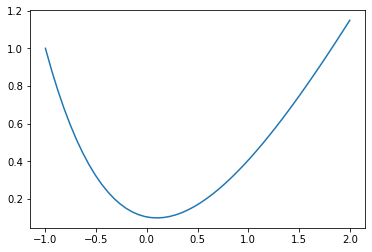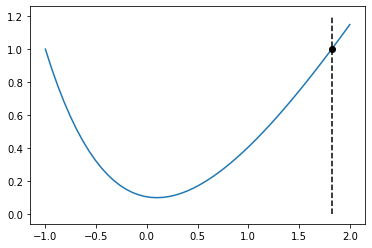# Solving First Order ODEs with Python¶

This notebook illustrates the the numerical solution of the general first order ODE using the most basic possible Python code. We'll need to import some functionality from the SciPy ecosystem:

In :
# A graphics library
import matplotlib.pyplot as plt

# Basic numerical tools
import numpy as np

# Scipy's numerical ODE solver
from scipy.integrate import odeint


The odeint command solves initial value problems (IVPs) of the form

$$y' = f(y,t), \: \: y(t_0) = y_0.$$

The command is acually a bit more general than that in that it works with systems of equations but we'll deal with that later.

To be more concrete, suppose that we wish to solve

$$y' = t-y, \: \: y(-1)=1, \: \text{ over } -1 \leq t \leq 1.$$

We can do so as follows:

In :
def f(y,t):
return t-y
t = np.linspace(-1,2)
y = odeint(f, 1, t).flatten()
y

Out:
array([1.        , 0.88306071, 0.77670215, 0.680296  , 0.59325121,
0.51501185, 0.44505495, 0.38288864, 0.32805026, 0.28010461,
0.23864236, 0.20327846, 0.17365072, 0.14941852, 0.13026143,
0.11587802, 0.10598482, 0.10031515, 0.09861818, 0.10065799,
0.10621266, 0.11507344, 0.127044  , 0.14193964, 0.15958666,
0.17982166, 0.20249094, 0.22744993, 0.25456265, 0.28370121,
0.31474528, 0.3475817 , 0.38210403, 0.41821215, 0.45581188,
0.49481463, 0.53513709, 0.57670088, 0.61943227, 0.66326194,
0.70812465, 0.75395905, 0.80070743, 0.84831553, 0.89673226,
0.94590963, 0.99580245, 1.04636824, 1.09756702, 1.14936121])

We can then visualize the solution:

In :
plt.plot(t,y)

Out:
[<matplotlib.lines.Line2D at 0x11b896a60>]Now suppose that we would like to know when the solution hits $y(t)=1$. We'll apply the SciPy solver called brentq to an interpolated version of the solution. First, we import a couple more libraries:

In :
from scipy.interpolate import interp1d
from scipy.optimize import brentq


We can now solve the problem as follows:

In :
y_func = interp1d(t,y)
t1 = brentq(lambda t: y_func(t)-1,1,2)
t1

Out:
1.8214088764185652

And visualize:

In :
plt.plot(t,y)
plt.plot([t1,t1],[0,1.2],'k--')
plt.plot(t1,1,'ko')

Out:
[<matplotlib.lines.Line2D at 0x11bbce220>]